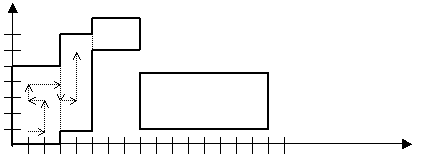ENG  RUS Timus Online JudgeOnline Judge
Problems
Authors
Online contests
About Online Judge
Frequently asked questions
Site news
Webboard
Links
Problem set
Submit solution
Judge status
Guide
Register
Update your info
Authors ranklist
Current contest
Scheduled contests
Past contests
Rules

Contest is over

## C. Rectangles Travel

Time limit: 1.0 second
Memory limit: 64 MB
On an endless sheet of checked paper there are axes of coordinates. A unit for measurement in this coordinates system is the length of the square’s edge. Also there are N rectangles on this sheet of paper. Their edges are parallel to the coordinate axis, and go through the borders between the squares. If we denote the coordinates of the lower left corner of the i-th rectangle with (xi, yi), and the coordinates of its upper right corner with (xi, yi), we will see, that:
x1 = 0, y1 = 0
xi = xi + 1
2 ≤ xixi ≤ 100
2 ≤ yiyi ≤ 100
If two rectangles with numbers i and i + 1 overlap, their common border disappears:A traveler starts his way from the point with the coordinates (1, 1), which, as it follows from the rules above, certainly lays in the first rectangle. The traveler walks strictly along the edges of the squares. He is not allowed to walk on the borders of the rectangles. Thus, he can leave one rectangle for another only through their disappeared common border. There is an example of some beginning of his walk on the picture.
The traveler’s goal is the point (xn − 1, yn − 1), which is obviously situated inside the last rectangle.

### Input

In the first line there is a positive integer n, 0 < n < 100000 — the number of rectangles on the plane. Then n lines follow, each one of them containing four integer numbers xi, yi, xi, yi, separated with spaces, satisfying the above rules.

### Output

You should output the length (the measurement unit is the edge of one square) of the shortest possible route for the traveler to go from the point (1, 1) to the point (xn − 1, yn − 1), or the number −1, if there exists no such route (the latter possibility is realized, for instance, on the picture).

### Sample

inputoutput
```2
0 0 3 5
3 1 5 7```
`8`
Problem Author: Leonid Volkov
Problem Source: USU Internal Contest, March 2002
To submit the solution for this problem go to the Problem set: 1202. Rectangles Travel